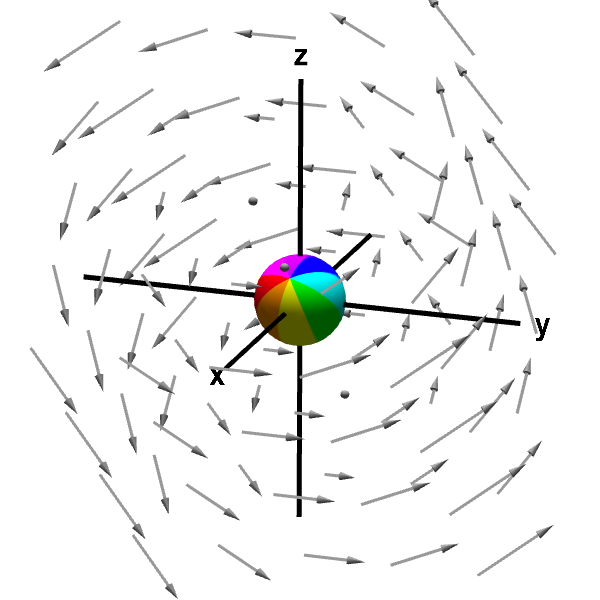# Math Insight

### Applet: A sphere rotated by a rotating vector fieldThe sphere embedded in this vector field is rotated by the swirling vector field, while its center is fixed at a point (originally the origin). The rotation of the sphere by the vector field is an indication of the vector field's curl. You can move the point at which the sphere is anchored by dragging it with your mouse.

This vector field is $\dlvf(x,y,z)= (-y,x-z,y).$ Its curl is \begin{align*} \curl \dlvf(x,y,z) &= \left(\pdiff{}{y}y-\pdiff{}{z}(x-z), \pdiff{}{z}(-y)- \pdiff{}{x}y, \pdiff{}{x}(x-z) - \pdiff{}{y}(-y) \right)\\ &=(2,0,2), \end{align*} which is a constant independent of point $(x,y,z)$ and always points at an angle halfway between the positive $x$ and $z$ axes. Note that $\curl \dlvf$ is parallel with the axis of rotation of the sphere. Since the curl is constant independent of the point $(x,y,z)$, the sphere spins in the same direction and with the same speed, no matter where it is moved.

You can rotate the graph with your mouse to better visualize the three-dimensional perspective.